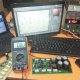Breaking News
Home / how to make inverter / How do you make a step up transformer?

# How do you make a step up transformer?

## How do you make a step up transformer?

Here in this post, we can learn How do you make a step up transformer. we can use 12 voltage battery to our 1000 Watts amplifier.normally we cannot use Battery to our amplifier. because our amplifier starts from 35-0-35 voltage. but here in this article, we can learn how to use the battery. how to make convert voltage 12 to 35 or higher. we are flowing the inverter system. we use a step up transformer to increase the voltage. let’s see what’s we are using to invert the voltage.step up transformer and step down transformer making rules are same. here we can see the bobbin. for an example, your bobbin is 4X4=16cm. we know that transformer calculation rule is 42. so 42/16=2.6. 2.6 turn need for 1 voltage. so we need 12-0-12 volt. 2.6X12=31.2 turn need in double.  now for the secondary. we already count that for 1 volt need 2.6 turns. so we need 40-0-40 voltage. 2.6X40=104 turn need in double.

Let’s watch the video, How to make the step up transformer

In this post, we can learn that how to make the transformer. in our next post, we can learn how to make the circuit diagram.

If you like our post please share and like.

transistor circuit diagram of 2sa1943 and 2sc5200

Thanks a lot to be with us. If you want another post then please visit our website.

We have another post for you. like as repairing amplifier,